Reach Us+44-1522-440391
A Joint Modeling Approach for Right Censored High Dimensional Multivariate Longitudinal Data | OMICS International
Journal of Biometrics & Biostatistics

Like us on:

All submissions of the EM system will be redirected to Online Manuscript Submission System. Authors are requested to submit articles directly to Online Manuscript Submission System of respective journal.

# A Joint Modeling Approach for Right Censored High Dimensional Multivariate Longitudinal Data

Miran A Jaffa1*, Mulugeta Gebregziabher2 and Ayad A Jaffa3,4

1Epidemiology and Population Health Department, Faculty of Health Sciences, American University of Beirut, Beirut, Lebanon, P.O.Box 11-0236 Riad El-Solh/Beirut, Lebanon 1107 2020, Lebanon

2Department of Public Health Sciences, Medical University of South Carolina, Charleston, South Carolina, 135 Cannon Street, Suite 303, Charleston, SC 2942., USA

3Department of Biochemistry and Molecular Genetics, Faculty of Medicine, American University of Beirut, Beirut, Lebanon, P.O.Box 11-0236 Riad El-Solh/Beirut, Lebanon 1107 2020, Lebanon

4Department of Medicine, Medical University of South Carolina, Charleston, SC 29425, USA

*Corresponding Author:
Miran A Jaffa
Epidemiology and Population Health Department
Faculty of Health Sciences, American University of Beirut
Beirut, Lebanon, P.O.Box 11-0236 Riad El-Solh/Beirut
Lebanon 1107 2020. Lebanon
Tel: +961-1-350000 Ext: 4603
Fax: +961-1-744470
E-mail: [email protected]

Received date: July 02, 2014; Accepted date: July 27, 2014; Published date: July 30, 2014

Citation: Jaffa MA, Gebregziabher M, Jaffa AA (2014) A Joint Modeling Approach for Right Censored High Dimensional Multivariate Longitudinal Data. J Biomet Biostat 5:203. doi: 10.4172/2155-6180.1000203

Copyright: © 2014 Jaffa MA, et al. This is an open-access article distributed under the terms of the Creative Commons Attribution License, which permits unrestricted use, distribution, and reproduction in any medium, provided the original author and source are are credited.

Visit for more related articles at Journal of Biometrics & Biostatistics

#### Abstract

Analysis of multivariate longitudinal data becomes complicated when the outcomes are of high dimension and informative right censoring is prevailing. Here, we propose a likelihood based approach for high dimensional outcomes wherein we jointly model the censoring process along with the slopes of the multivariate outcomes in the same likelihood function. We utilized pseudo likelihood function to generate parameter estimates for the population slopes and Empirical Bayes estimates for the individual slopes. The proposed approach was applied to jointly model longitudinal measures of blood urea nitrogen, plasma creatinine, and estimated glomerular filtration rate which are key markers of kidney function in a cohort of renal transplant patients followed from kidney transplant to kidney failure. Feasibility of the proposed joint model for high dimensional multivariate outcomes was successfully demonstrated and its performance was compared to that of a pairwise bivariate model. Our simulation study results suggested that there was a significant reduction in bias and mean squared errors associated with the joint model compared to the pairwise bivariate model.

#### Keywords

Informative right censoring; Joint modelling; Likelihood based approach; Multivariate longitudinal outcomes; Random effect; Slope estimation

#### Introduction

Kidney function is assessed using the markers serum creatinine, blood urea nitrogen (BUN), and estimated glomerular filtration rate (eGFR). All three markers are needed to assess kidney function since each marker has its own limitation. For instance, serum creatinine varies inversely with glomerular filtration rate (GFR) and creatinine levels are influenced by age and gender . Whereas, BUN levels could fluctuate with protein intake, catabolism and tubular reabsorption of urea  and eGFR could be less accurate in obese individuals and those with normal or near normal GFR [3-5]. Also in most clinical settings the exact values of BUN, creatinine and eGFR provide little information on disease severity. What is more important is monitoring the rate of change in serum creatinine, BUN and eGFR over time to determine disease progression or to ascertain if state of disease is stable or changing . This is done by taking repeated measures of these markers on the same patient and by calculating the rate of change or slopes for each of these markers to provide an evaluation of disease progression over time. This is critically significant for patients who undergo kidney transplant where a routine follow-up evaluation for their kidney function is mandated to determine how well their kidneys are functioning post-transplantation and to verify if graft failure is likely to transpire. For the cohort of renal transplantation we considered in this study, longitudinal measures of creatinine and BUN are recorded and eGFR levels are computed post transplant repeatedly over time till patient experiences renal graft failure. Patients who experience graft failure will therefore have an incomplete set of repeated measures on their creatinine, BUN and eGFR, a situation referred to as informative right censoring.

Slope estimation for these outcomes is complicated in the presence of informative right censoring and special method of analysis that accounts for this problem should be conducted so that valid inferences are reached. If this type of censoring is ignored or treated as noninformative, it could result in biased estimates and lead to inaccurate inferences . Since informative right censoring is widespread in longitudinal studies several statistical methods were developed for slope estimation that account for right censoring [8-16]. However, all these methodological approaches have been developed for a single longitudinal outcome with informative right censoring. Although multiple outcomes are commonly encountered in medical research setting methodological approaches for slope estimation for multivariate longitudinal outcomes with informative right censoring are still not well developed . This paucity is due to the level of complexity that accompanies such approaches where informative right censoring and the different correlations should be adjusted for . This problem becomes much more compounded when the outcomes are of high dimension which results in an increase in the number of parameters in the variance-covariance matrix and in the number of estimates, and hence could lead to convergence problems in many situations. Few studies have developed methods for slope estimation for bivariate longitudinal outcomes adjusting for informative right censoring [19-21]. For example, a joint model for a time to clinical event and for repeated measures over time on surrogate outcomes was presented by Xu and Zeger [22,23]. In this study a multivariate mixed model was used for the joint analysis of multivariate repeated measures data and times to an event with an underlying assumption of conditional independence between the censoring time and the biomarkers given the latent process for each outcome. A pairwise fitting model was proposed to analyze multivariate outcomes [24,25]; but this approach could lead to efficiency loss. He and Luo developed a joint model of the multilevel item response theory (MLIRT) and Cox’s proportional hazard model for the time to the dependent terminal event with shared random effects to link the two models .

Joint modeling and maximization of the full multivariate likelihood function if feasible is a favorable approach . However, this approach becomes difficult to implement with high dimensional outcomes given the computational complexity and convergence problem that could be encountered in such a setting. In the current article we aim to extend the bivariate model developed by Jaffa et al.  to high dimensional multivariate outcomes and to demonstrate its implementation feasibility through its successful convergence. Specifically, we propose a likelihood based approach where we jointly model the censoring process along with the slopes of the multivariate longitudinal outcomes and their variance-covariance matrix in the same multivariate likelihood function. This likelihood function is then maximized to generate slope estimates for the population as well as individual subjects. Estimating the individual slopes provides an assessment for the rate of change for every subject and therefore a case by case prognosis of the disease. This approach accounts for the informative right censoring and the correlation between the longitudinal outcomes. Specifically, the number of visits (before censoring happens due to kidney failure) for every patient is modeled using a discrete probability model with the individual slopes of the outcomes as covariates in the model. The innovation in the proposed model resides in its feasibility to handle high dimensional outcomes by jointly modeling all the slopes, their correlations and the censoring process in one likelihood function and casting the problem in such a way that standard software could be used to generate estimates for multivariate longitudinal outcomes of high dimension. We first used simulated data to assess the performance of the proposed method in terms of bias and efficiency and made comparison with the bivariate approach proposed in Jaffa et al.  applied in a pairwise fashion. This comparison enables us to determine if incorporating all the correlations concomitantly in the same likelihood function leads to a better precision than that of the bivariate modeling with pairwise joint modeling of the correlations. Moreover, a small simulation study was conducted on outcomes with different dimensions (7 outcomes and 10 outcomes) to confirm the feasibility of the model for high dimensional data. We then used data from a cohort of renal transplant patients at the Medical University of South Carolina where the markers of interest: creatinine, BUN and eGFR, are measured for each patient in a longitudinal fashion . The objective of this study is to assess kidney function over time by estimating the population and individual slopes corresponding to these kidney markers. The baseline measures for BUN, creatinine, and eGFR recorded prior to the transplantation do not have any impact on kidney function after the transplantation since a new organ is transplanted and post operative measures of these markers determine the progression of disease. This is demonstrated by Kaplan Meier survival analysis which confirmed that there is no significant difference in survival between the group of patients whose baseline pre-transplant eGFR levels is less than 15 ml/min/1.73 m2 and those who are above this cutoff point (P-value = 0.5). Those with less than 15 ml/min are patients with kidney failure while those with more than 15 correspond to those who have severe to mild kidney damage prior to transplantation. This classification of patients is based on that of Perazella and Reilly . Failing to capture a significant difference in survival between the two groups of patients indicates that the pre-transplant baseline eGFR levels do not affect kidney function post-transplantation and intercept could therefore be discarded in such a clinical setting and the corresponding statistical model could focus on the slopes only.

#### Multivariate Model

The multivariate model proposed in this manuscript is an extension of the bivariate approach . Specifically the multivariate model could be specified as follows:

Consider a set of i=1,…,n independent subjects, with k=1,…,q multivariate correlated outcomes (yij1, yij2,…, yijq) recorded at j=1,..,p predetermined times denoted as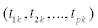. These p times are not necessarily equally spaced. Because of right censoring, the ith individual has mi number of visits or observations, where mi ≤ p, corresponding to times of measurement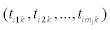. We assume that (yij1, yij2,…, yijq) are all observed at all time points before dropout, i.e., the q outcomes are either all measured or all are missing. For example in the renal transplantation example, a patient has measurements on BUN, creatinine, and eGFR till renal failure. In this case measurements are no longer taken on any of the outcomes after failure.

The underlying model for (yij1, yij2,…, yijq) at time tik is assumed to be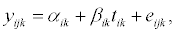(1)

for the ith individual, jth observation and kth outcome, with random effects αik and βik, and random error eijk which is assumed to be normal with mean zero and variance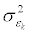. We note here that, in a model with informative right censoring, the probability of being censored depends on unobserved data. In our informative censoring model, the probability of being censored depends on the random slopes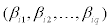, that will be incorporated in the likelihood function described later in this section. Specifically, our interest is in estimating multivariate population slopes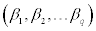and predicting the multivariate individual random effects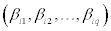that are assumed to be correlated with covariance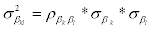and follow a multivariate normal distribution: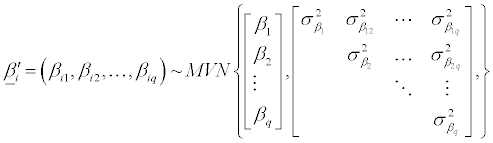(2)

Hence by having the slopes of the outcomes correlated and incorporating these correlations in the likelihood function (described below), we are therefore accounting for the correlations between the outcomes. Moreover, since interest is in the slopes, the individual’s observations yijk pertaining for very outcome are reduced to the generalized least square estimates (GLS) denoted as bik that are obtained using SAS proc mixed. The likelihood is a function of the following:

Assumption 1: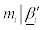having Truncated Discrete Distribution with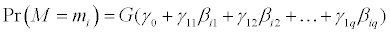(3)

This assumption states that the number of observations for each individual follows a discrete probability distribution with probability dependent on the individual slopes. Also, γ101112,… ,γ1q are the parameters of the censoring distribution. This discrete censoring distribution can be right-truncated since the study may terminate before observing the withdrawal of all the subjects and left truncated since at least two observations need to be recorded for every individual so that individual slopes can be estimated. A special case of the discrete censoring distribution is the truncated geometric distribution.

Assumption 1 can then be defined as follows:~ Truncated geometric with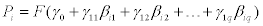(4)

with probability model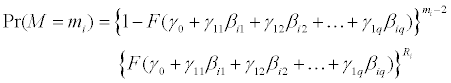(5)

With mi = 2,3,…,p; p is the number of prespecified measurement time points and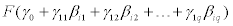is a function of individual slopes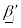. To account for right truncation the geometric distribution was modified by introducing an indicator variable denoted as Ri to the geometric probability function. The indicator variable Ri=1 if censoring occurred and Ri=0 otherwise. In a previous study  it was suggested that logistic model may be employed for the sake of simplicity and the functionis therefore defined as follows: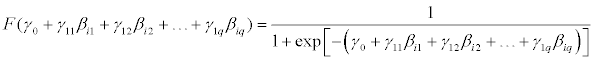(6)

Note that if censoring parameters γ1112,…,γ1q are all equal to zero then the drop-out process does not depend on any of the outcomes and is therefore non-informative. In case of dropout, it is assumed that no more measurements are recorded on all the outcomes. Thus each subject will have the same number of observations for all q outcomes.

Assumption 2:

Given mi, the observed individuals’ GLS estimates bik for every outcome are assumed to be normally distributed with mean βik and variance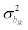The censoring process is viewed as informative or non-ignorable in the sense that the censoring mechanism is dependent on the unobserved random vector. In this context,is both a parameter in the distribution of the vector of GLS estimates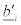and is unobserved itself. The marginal likelihood, integrated over the unobserved random effectsis maximized to obtain the maximum likelihood estimates for the population slopes and censoring parameters, and empirical Bayes estimates for the individual slopes. The joint distribution of mi, andis used in the likelihood function as follows: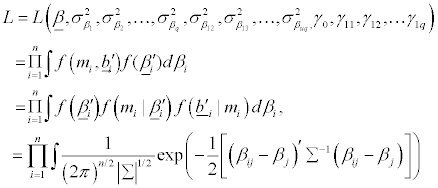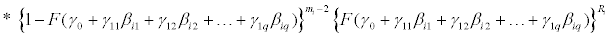(7)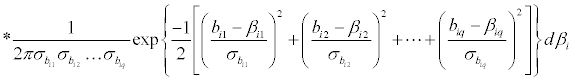The log of this likelihood is maximized to obtain estimates of the population slopes (β12,…,βq) in addition to the parameters of the dropout model (γ011121q). The Empirical Bayes estimates of the individual random slopes (βi1i2,…,βiq) are obtained via the approach described . The slopesof the q multivariate longitudinal outcomes are assumed to follow a multivariate normal distribution and the correlations between these slopes are included in the variancecovariance matrix denoted as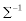and hence are incorporated in the likelihood function.

SAS procedure NLMIXED (SAS 9.3 Institute Inc.) is used to implement the proposed approach .

#### Simulation Study

A simulation study was conducted to assess the performance of the multivariate model with three outcomes in comparison to the bivariate model described by Jaffa  assuming geometric distribution for the patients’ number of visits. Performance was determined by bias, and means squared errors for the population and individual slopes denoted as MSEa and MSEb respectively and evaluated as follows: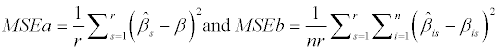(8)

with n being the number of subjects in each data set and r being the total number of replications. The bivariate model was used in a pairwise fashion for all possible pairwise combination of outcomes. The average of bias and that of MSEa and MSEb were computed respectively for every outcome. In the proposed multivariate model the slopes of the three outcomes and their correlations were all concurrently incorporated in the same likelihood function which is maximized to obtain the slopes estimates. This simulation study enables us to determine whether maximizing the multivariate likelihood function which incorporates all the slopes for the outcomes on which the censoring process depends has any effect on the accuracy of the estimates compared to the bivariate model that accounts for only two of these outcomes and ignores the rest. Specifically this comparison enables us to determine whether incorporating all slopes simultaneously in the likelihood function has any effect on the precision and accuracy of the slope estimates assessed by bias and mean squared errors compared to the pairwise bivariate model. The number of visits per individual was randomly generated from the truncated geometric distribution that depended on three censoring parameters γ1112, and γ13. The number of observations ranged from 2 to 7 recorded at prespecified time points (tij) 0, 1, 3, 6, 12, 24, and 36 months and a linear relationship was assumed between each outcome and log(tijk+1). Thus, the model for the outcomes is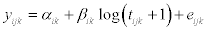for k=1,2,3. Errors eijk were assumed to be normally distributed with mean zero and variance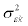. The parameters used in the simulation study were the ones acquired from the renal transplant with estimated slopes β1= βcreatinine=-0.089, β2BUN=-0.142, and β3eGFR=0.302, slope variances of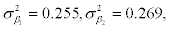and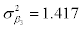, and error variances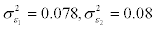and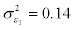. Different values of correlation coefficient between the three outcomes were considered ranging from low to high correlations and 2000 replications each with size of 200 were generated. In specific, low correlations with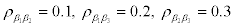,mid correlation with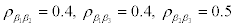and high correlation with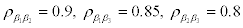were examined along with different censoring levels. Incorporating different levels of correlation between the outcomes and allowing the censoring process to vary in magnitude help us understand the impact of the correlation and censoring on the accuracy of the estimates.

Tables 1-3 present the bias and mean square errors for the slopes estimates for the three outcomes under the trivariate and bivariate models applied in a pairwise fashion. The reported means for bias for the first outcome in the bivariate model correspond to the mean of bias associated with the bivariate outcomes one and two (first pair), and one and three (second pair). The same computation was followed for the bias for outcomes two and three. Mean MSEa and mean MSEb for the bivariate model were computed similarly to the mean bias. We will start first by discussing the results reported in Table 1 wherein low correlations between the outcomes were assumed. In this context, when the censoring level was low (γ1=3.5, γ2=2.7 and γ3=3.0) bias for the three outcomes was decreased by 52% on average under the multivariate model compared to the pairwise bivariate model, MSEa by 23% and MSEb by 54%. When the censoring level increased to a midlevel with γ1=4.2, γ2=4.8 and γ3=5.1 bias decreased on average for the three outcomes by 54%, MSEa by 10% and MSEb by 26% for the multivariate model compared to the pairwise bivariate model. When the censoring level increased to high censoring with γ1=7.8, γ2=7.5 and γ3=7.2 bias decreased by 26%, MSEa by 41% and MSEb by 20%. These results indicate that regardless of the censoring levels the estimates generated under the multivariate model had better precision and accuracy compared to the bivariate model. When the correlation levels between the outcomes increased to mid levels with ρ12=0.4, ρ13=0.4, and ρ23=0.5 (Table 2), bias across all levels of censoring decreased by 40%, MSEa by about 15% and MSEb by 28% for multivariate compared to bivariate model and this decrease was demonstrated across all levels of censoring. Similar results were observed with high correlation levels of ρ12=0.9, ρ13=0.85, and ρ23=0.8 (Table 3). In this context, under the multivariate model we observed a decrease in bias, MSEa and MSEb by an average of 40%, 15% and 20% respectively. These results suggest that regardless of the correlation and censoring levels modeling all outcomes simultaneously in the likelihood function and accounting for the correlations among them reduced the bias and MSEs associated with slope estimates and thus increased accuracy of estimation in comparison to modeling only two of the outcomes and ignoring the rest. Feasibility of the model to fit high dimensional outcomes was also verified via a small simulation study conducted at a high level of censoring. In this context we showed successful convergence of our model and slope estimates for 7 outcomes were attained with average bias of 0.006, MSEa of 0.0017 and MSEb of 0.012, as well as those for 10 outcomes with associated average bias of 0.008, MSEa of 0.0097, and MSEb of 0.059. Thus, joint modeling of multivariate slope outcomes, their correlations, and the censoring process was implemented and convergence on different dimensions of the outcomes was successfully achieved.

Parameter Trivariate model Bivariate model
γ1♣=3.5, γ2♣=2.7, γ3♣=3.0 Bias_1*100
Bias_2*100
Bias_3*100
MSEa_1*103
MSEa_2*103
MSEa_3*100
MSEb_1*100
MSEb_2*10
MSEb_3*100
-0.007
-0.028
-0.119
0.144
0.853
0.127
0.102
0.091
0.215
-0.021
-0.076
0.163
0.205
0.909
0.187
0.113
0.094
0.567
γ1♣=4.2, γ2♣=4.8, γ3♣=5.1 Bias_1*100
Bias_2*100
Bias_3*100
MSEa_1*103
MSEa_2*103
MSEa_3*100
MSEb_1*100
MSEb_2*10
MSEb_3*100
-0.012
-0.151
-0.132
0.140
0.805
0.154
0.104
0.097
0.266
0.022
0.343
0.346
0.150
0.856
0.173
0.116
0.110
0.611
γ1♣=7.8, γ2♣=7.5, γ3♣=7.2 Bias_1*100
Bias_2*100
Bias_3*100
MSEa_1*103
MSEa_2*103
MSEa_3*100
MSEb_1*100
MSEb_2*10
MSEb_3*100
-0.073
-0.303
-0.126
0.132
0.083
0.182
0.107
0.099
0.335
0.101
-0.310
-0.237
0.161
0.921
0.214
0.115
0.109
0.588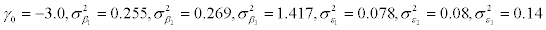1♣, first outcome; 2♣, second outcome; 3♣, third outcome; Bivariate model mean bias, mean MSEa, mean MSEb of the pairwise bivariate model estimates.

Table 1: Comparisons of the performance of the trivariate and bivariate models,Parameter Trivariate model Bivariate model†
γ1♣=3.5, γ2♣=2.7, γ3♣=3.0 Bias_1*100
Bias_2*100
Bias_3*100
MSEa_1*103
MSEa_2*103
MSEa_3*100
MSEb_1*100
MSEb_2*10
MSEb_3*100
-0.011
0.015
0.041
0.133
0.797
0.125
0.103
0.098
0.219
0.015
0.141
0.144
0.146
0.846
0.169
0.112
0.111
0.593
γ1♣=4.2, γ2♣=4.8, γ3♣=5.1 Bias_1*100
Bias_2*100
Bias_3*100
MSEa_1*103
MSEa_2*103
MSEa_3*100
MSEb_1*100
MSEb_2*10
MSEb_3*100
-0.016
-0.204
-0.147
0.142
0.841
0.135
0.105
0.099
0.234
0.054
-0.265
-0.177
0.158
0.922
0.168
0.115
0.111
0.611
γ1♣=7.8, γ2♣=7.5, γ3♣=7.2 Bias_1*100
Bias_2*100
Bias_3*100
MSEa_1*103
MSEa_2*103
MSEa_3*100
MSEb_1*100
MSEb_2*10
MSEb_3*100
-0.114
-0.413
-0.158
0.133
0.899
0.129
0.105
0.102
0.228
-0.123
-0.513
-0.234
0.140
0.921
0.199
0.114
0.113
0.636

Table 2: Comparisons of the performance of the trivariate and bivariate models,Parameter Trivariatemodel Bivariate model†
γ1♣=3.5, γ2♣=2.7, γ3♣=3.0 Bias_1*100
Bias_2*100
Bias_3*100
MSEa_1*103
MSEa_2*103
MSEa_3*100
MSEb_1*100
MSEb_2*10
MSEb_3*100
-0.064
-0.078
-0.071
0.143
0.777
0.132
0.116
0.121
0.270
-0.107
-0.128
0.091
0.146
0.810
0.198
0.121
0.122
0.617
γ1♣=4.2, γ2♣=4.8, γ3♣=5.1 Bias_1*100
Bias_2*100
Bias_3*100
MSEa_1*103
MSEa_2*103
MSEa_3*100
MSEb_1*100
MSEb_2*10
MSEb_3*100
-0.055
-0.178
-0.048
0.139
0.839
0.148
0.119
0.124
0.264
-0.111
-0.443
-0.141
0.152
0.892
0.205
0.122
0.124
0.615
γ1♣=7.8, γ2♣=7.5, γ3♣=7.2 Bias_1*100
Bias_2*100
Bias_3*100
MSEa_1*103
MSEa_2*103
MSEa_3*100
MSEb_1*100
MSEb_2*10
MSEb_3*100
-0.135
-0.479
-0.216
0.140
0.801
0.165
0.120
0.119
0.317
-0.183
-0.648
-0.241
0.154
0.958
0.195
0.121
0.125
0.666

Table 3: Comparisons of the performance of the trivariate and bivariate models,#### Cohort of Renal Transplant

The multivariate model was illustrated using a cohort of renal transplantation at the Medical University of South Carolina. A total of 110 patients who underwent kidney transplant in the calendar year 2000 were followed and demographical information along with a three year repeated measures of their kidney function using the markers serum creatinine, BUN and eGFR were recorded. The normal values for creatinine are in the range of 0.7 to 1.3 mg per 100 ml of blood, for BUN are between 8 and 25 mg per 100 ml of blood and for eGFR is approximately 90 and 120 mL/min/1.73 m2 for men and women respectively. A decrease in eGFR and an increase in creatinine and BUN could be an indication of disease progression. Repeated measures on these markers were recorded between the years 2000 and 2003 yielding seven data points registered at baseline pre-transplantation (month 0), and post-transplantation at months 1, 3, 6,12, 24 and 36. Patients were followed at predetermined time points following a prespecified schedule for all outcomes and measurements were taken repeatedly until graft failure is encountered. In this situation, patients revert back to dialysis treatment and acquisition of their renal markers is no longer possible. This leads to patient dropout from the follow-up study resulting in 19% informative right censoring due to graft failure.

For every individual we use the baseline adjusted model by incorporating the baseline measure as a covariate in the model as such: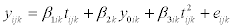where i=1,…,n, j=1,…, mi and k=1,…,q. In Figure 1a and 1b) we present the mean levels of BUN and eGFR, and those of creatinine respectively against follow-up months. A curvilinear relationship between follow-up months and each marker was shown, so in order to linearize it we log transformed and we introduced a quadratic term in the model’s equation. The logarithmic transformation linearized this relationship to a certain extent but not fully so we still needed to include the quadratic term.

Figure 1: BUN, eGFR (a), and Serum creatinine mean levels (b) respectively plotted against follow-up months.

The mean levels of the outcomes (BUN, eGFR, and creatinine) were plotted against the number of visits that range from 2 to 7 Figure 2a for BUN and eGFR and Figure 2b for creatinine. This figure shows that patients with high number of visits (5 and above) appear to have lower BUN and creatinine levels and higher eGFR levels on average compared to those with lower number of visits (below 5 visits). Since, the lower the creatinine and BUN, and the higher the eGFR the better the prognosis of kidney disease, this figure therefore indicates that the length of stay in the study measured by number of visits is determined by the levels of these markers.

Figure 2: BUN, eGFR (a), and Serum creatinine mean levels (b) respectively plotted against number of visits.

Our proposed multivariate model was applied to the renal transplant dataset, to estimate population and individual slopes pertaining to the renal markers creatinine, BUN and eGFR. We assumed that the number of visits follow a truncated geometric distribution. The adequately modeled using the specified geometric distribution with test statistics P-value=0.6, X2=0.3 DF=1. The estimated population slope for creatinine, BUN and eGFR were respectively βcreatinine=-0.089 (SE=0.0117), βBUN=-0.142 (SE=0.011), and βeGFR=0.302 (SE=0.0155) with P-value<0.0001. The estimated variance-covariance matrix ofwas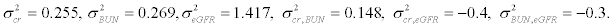The mean values of the estimated individual slopes were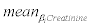of -0.086 ± 0.494,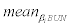of -0.20 ± 0.515, and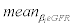of 0.348 ± 1.178. The censoring parameters for all three markers were γcreatinine=-0.093, γBUN=-0.0584, γeGFR=0.091 (P-value<0.0001). The significance in the censoring parameters for all three markers indicates that the censoring process was informative and significantly dependent on all three markers.

#### Discussion

In this article we propose a joint likelihood based approach for a multivariate mixed model that is an extension of the bivariate model by Jaffa et al. . The proposed multivariate model generates maximum likelihood estimates for the population slopes and empirical Bayes estimates for the individual slopes that are adjusted for informative right censoring. To account for this type of censoring a discrete distribution for the number of visits was assumed. Random slopes for the outcomes and the correlation between them were also concomitantly incorporated in the maximum likelihood function. The proposed multivariate model was applied to the cohort of renal transplant patients and the three biomarkers serum creatinine, BUN and eGFR that are typically measured to assess renal function over time following transplantation were modeled and their corresponding slopes were estimated. Moreover, individual slope for every patient was obtained thus making it viable to monitor disease progression on an individual and population basis. In this study we aimed at demonstrating the feasibility of the joint multivariate approach to fit the likelihood function for high dimensional outcomes (3, 7 and 10 outcomes) using standard software and successfully obtaining the population and individual slopes with minimal bias and MSEs for the different dimension of the multivariate outcomes. The model is not necessarily just limited to up to 10 outcomes but could be also extended to higher dimensions. Joint modeling approach proposed in this research work has always been considered advantageous yet challenging to implement compared to other methods such as pairwise model fitting proposed to handle situations of high dimensional outcomes. Specifically, our proposed joint modeling approach is favored over the pairwise model fitting since it increases efficiency and accuracy of the estimates . In this context, our simulation study showed that fitting the full multivariate model had negligible bias and mean squared errors associated with slope estimates and that accuracy of estimates increased with this approach compared to the pairwise bivariate model. Joint modeling of the multivariate outcomes has its established advantages compared to the univariate separate analysis. The latter approach ignores the intrinsic correlations between the outcomes while the multivariate joint analysis exploits this correlation to generate more accurate estimates, and controls for type 1 error that might emanate from the univariate analysis when conducted without accounting for multiple comparisons . Moreover, we have recently shown that joint bivariate analysis results in more precise estimates compared to the univariate separate analysis and the same conclusion should follow in the multivariate setting . In a recent study, Jaffa conducted a sensitivity analysis on this model and it was shown that the proposed model is robust for assumptions about the underlying distributions for the number of visits mi . In this regard, an average increase of 18% was detected for bias and MSEs when the underlying distribution of the number of visits was mispecified and wrong assumptions were considered. In addition, we were also able to verify that violation of the normality assumption for the outcomes had a minor effect on the accuracy of the estimates and less than 10% increase in bias and MSEs was captured for the non-normal compared to normal outcomes. This robustness to assumptions misspecification makes it plausible for the proposed model to be applied to a wider range of datasets with multivariate high dimensional longitudinal outcomes.

#### Acknowledgement

This work was supported by the National Institutes of Health Grants HL077192 and HL087986 (AAJ).

#### References

Select your language of interest to view the total content in your interested language

### Article Usage

• Total views: 12089
• [From(publication date):
August-2014 - May 27, 2019]
• Breakdown by view type
• HTML page views : 8237
• PDF downloads : 3852

## Post your commentCan't read the image? click here to refresh
###### Peer Reviewed Journals

Make the best use of Scientific Research and information from our 700 + peer reviewed, Open Access Journals

International Conferences 2019-20

Meet Inspiring Speakers and Experts at our 3000+ Global Annual Meetings

Top